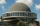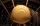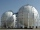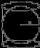# Sphere + body volume - math problems

1. KostkaKostka je vepsána do koule o poloměru r = 6 cm. Kolik procent tvoří objem kostky z objemu koule?
2. Wall thicknessThe hollow metal ball has an outside diameter of 40 cm. Determine the wall thickness if the weight is 25 kg and the metal density is 8.45 g/cm3.
3. Hemisphere - roofThe shape of the observatory dome is close to the hemisphere. Its outer diameter is 11 m. How many kilograms of paint and how many liters of paint is used for its double coat if you know that 1 kg of paint diluted with 1 deciliter of paint will paint an a
4. The ballThe ball has a radius of 2m. What percentage of the surface and volume is another sphere whose radius is 20% larger?
5. The prison ballCalculate the density of the material that the prison ball is made from if you know its diameter is 15cm and its weight is approximately 2.3kg. With the help of mathematical-physicochemical tables estimate what material the ball is made from.
6. The hemisphereThe hemisphere container is filled with water. What is the radius of the container when 10 liters of water pour from it when tilted 30 degrees?
7. Alien shipThe alien ship has the shape of a sphere with a radius of r = 3000m, and its crew needs the ship to carry the collected research material in a cuboid box with a square base. Determine the length of the base and (and height h) so that the box has the large
8. PebbleThe aquarium with internal dimensions of the bottom 40 cm × 35 cm and a height of 30 cm is filled with two-thirds of water. Calculate how many millimeters the water level in the aquarium rises by dipping a pebble-shaped sphere with a diameter of 18 cm.
9. Sphere VSFind the surface and volume of a sphere that has a radius of 2 dm.The radius of the sphere we reduce by 1/3 of the original radius. How much percent does the volume and surface of the sphere change?
11. Sphere floatingWill float a hollow iron ball with an outer diameter d1 = 20cm and an inside diameter d2 = 19cm in the water? The iron density is 7.8 g/cm 3. (Instructions: Calculate the average sphere density and compare with the density of the water. )
12. Iron ballThe iron ball has a weight of 100 kilograms. Calculate the volume, radius, and surface if the iron's density is h = 7.6g/cm3.
13. Volume of ballFind the volume of a volleyball that has a radius of 4 1/2 decimeters. Use 22/7 for π
14. Spherical tankThe tank of a water tower is a sphere of radius 35ft. If the tank is filled to one quarter of full, what is the height of the water?
15. Cube into sphereThe cube has brushed a sphere as large as possible. Determine how much percent was the waste.
16. Hemispherical hollowThe vessel hemispherical hollow is filled with water to a height of 10 cm =. How many liters of water are inside if the inside diameter of the hollow is d = 28cm?
17. Equilateral cylinderA sphere is inserted into the rotating equilateral cylinder (touching the bases and the shell). Prove that the cylinder has both a volume and a surface half larger than an inscribed sphere.
18. InscribedCube is inscribed in the cube. Determine its volume if the edge of the cube is 10 cm long.
19. Cube, cuboid, and sphereVolumes of a cube and a cuboid are in ratio 3: 2. Volumes of sphere and cuboid are in ratio 1: 3. In what ratio are the volumes of cube, cuboid, and sphere?
20. Inscribed sphereHow many percents of the cube volume takes the sphere inscribed into it?

Do you have an interesting mathematical word problem that you can't solve it? Enter it, and we can try to solve it.

We will send a solution to your e-mail address. Solved examples are also published here. Please enter the e-mail correctly and check whether you don't have a full mailbox.

Please do not submit problems from current active competitions such as Mathematical Olympiad, correspondence seminars etc...